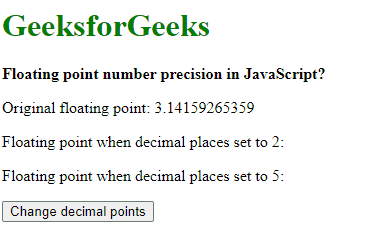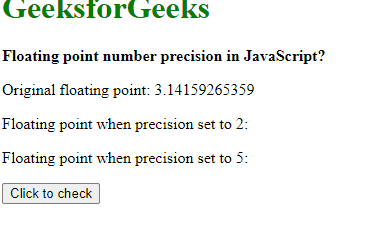# Floating point number precision in JavaScript

The representation of floating points in JavaScript follows the IEEE-754 format. It is a double precision format where 64 bits are allocated for every floating point. The displaying of these floating values could be handled using 2 methods:

Using toFixed() Method: The number of decimal places in float values can be set using the toFixed() method. This method converts the number into a string, keeping the specified number of digits after the point. If no value is passed as a parameter, then it takes 0 as default value i.e. no decimal points are displayed.

Syntax:

`number.toFixed(digits)`

Example:

## html

 `<``h1` `style``=``"color: green"``> ` `    ``GeeksforGeeks ` ` ` ` `  `<``b``> ` `    ``Floating point number precision ` `    ``in JavaScript? ` ` ` ` `  `<``p``> ` `    ``Original floating point: 3.14159265359 ` ` ` ` `  `<``p``> ` `    ``Floating point when decimal places set ` `    ``to 2: <``span` `class``=``"output-2"``> ` ` ` ` `  `<``p``> ` `    ``Floating point when decimal places set ` `    ``to 5: <``span` `class``=``"output-5"``> ` ` ` ` `  `<``button` `onclick``=``"setDecimalPlaces()"``> ` `    ``Change decimal points ` ` ` ` `  `<``script` `type``=``"text/javascript"``> ` `    ``function setDecimalPlaces() { ` `        ``pi = 3.14159265359; ` `        ``twoPlaces = pi.toFixed(2); ` `        ``fivePlaces = pi.toFixed(5); ` `     `  `        ``document.querySelector('.output-2').textContent ` `                ``= twoPlaces; ` `        ``document.querySelector('.output-5').textContent ` `                ``= fivePlaces; ` `    ``} ` ``

Output:Floating point number precision in JavaScript

Using toPrecision() Method: The number of total digits in float values can be set using the toPrecision() method. This method converts the number into a string, keeping the total number of digits of the value as specified and rounding them to the nearest number. If no value is passed as a parameter, then the function acts as a toString() function effectively returning the value passed as a string.

Syntax:

`number.toPrecision(precision)`

Example:

## html

 `<``h1` `style``=``"color: green"``> ` `    ``GeeksforGeeks ` ` ` ` `  `<``b``> ` `    ``Floating point number precision ` `    ``in JavaScript? ` ` ` ` `  `<``p``> ` `    ``Original floating point: 3.14159265359 ` ` ` ` `  `<``p``> ` `    ``Floating point when precision set to ` `    ``2: <``span` `class``=``"output-2"``> ` ` ` ` `  `<``p``> ` `    ``Floating point when precision set to ` `    ``5: <``span` `class``=``"output-5"``> ` ` ` ` `  `<``button` `onclick``=``"setPrecision()"``> ` `    ``Click to check ` ` ` ` `  `<``script` `type``=``"text/javascript"``> ` `    ``function setPrecision() { ` `        ``pi = 3.14159265359; ` `        ``twoPlaces = pi.toPrecision(2); ` `        ``fivePlaces = pi.toPrecision(5); ` `     `  `        ``document.querySelector('.output-2').textContent ` `                ``= twoPlaces; ` `        ``document.querySelector('.output-5').textContent ` `                ``= fivePlaces; ` `    ``} ` ``

Output:Floating point number precision in JavaScript

Whether you're preparing for your first job interview or aiming to upskill in this ever-evolving tech landscape, GeeksforGeeks Courses are your key to success. We provide top-quality content at affordable prices, all geared towards accelerating your growth in a time-bound manner. Join the millions we've already empowered, and we're here to do the same for you. Don't miss out - check it out now!

Previous
Next